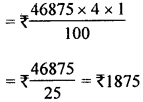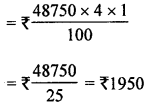## ML Aggarwal Class 8 Solutions for ICSE Maths Chapter 8 Simple and Compound Interest Ex 8.2

Question 1.
Calculate the compound interest on ₹6000 at 10% per annum for two years.
Solution:
Rate of interest = 10% per annum
Principal for the first year = ₹6000
Interest for the first year = ₹$$\frac{6000 \times 10 \times 1}{100}$$ = ₹6000
Amount at the end of first year = ₹6000 + ₹600 = ₹6600
Principal for the 2nd year = ₹6600
Interest for the 2nd year = ₹$$\frac{6600 \times 10 \times 1}{100}$$ = ₹660
Amount for the second year = ₹6600 + ₹660 = ₹7260
∴ Compound interest for 2 years = final amount – (original) Principal
= ₹ 7260 – ₹6000 = ₹1260

Question 2.
Salma borrowed from Mahila Samiti a sum of ₹ 1875 to purchase a sewing machine. If the rate of interest is 4% per annum, what is the compound interest that she has to pay after 2 years?
Solution:
Principal for the 1st year = ₹1875
Rate of’interest = 4% p.a.
Interest for the 1st year = ₹ $$\frac{6000 \times 10 \times 1}{100}$$ = ₹600
Amount at the end of first year = ₹1875 + ₹75 = ₹1950
Principal for the 2nd year = ₹1950
Interest for the 2nd year = ₹ $$\frac{6000 \times 10 \times 1}{100}$$ = ₹600
Amount at the end of 2nd year = ₹1950 + ₹78 = ₹2028
∴ Compound interest paid by Salma
= Final amount – (original) Principal
= ₹2028 – ₹1875 = ₹153

Question 3.
Jacob invests ₹12000 for 3 years at 10% per annum. Calculate the amount and the compound interest that Jacob will get after 3 years.
Solution:
Principal for the 1st year = ₹ 12000
Rate = 10% p.a.
Interest for the 1st year = ₹ $$\frac{12000 \times 10 \times 1}{100}$$ = ₹1200
Amount at the end of 1 st year = ₹12000 + ₹1200 = ₹13200
Principal for the 2nd year = ₹ 13200
Interest for the 2nd year = ₹ $$\frac{13200 \times 10 \times 1}{100}$$ = ₹ 1320
Amount at the end of 2nd year = ₹13200 + ₹1320 = ₹14520
Principal for the 3rd year = ₹ 14520
Interest for the 3rd year = ₹ $$\frac{14520 \times 10 \times 1}{100}$$ = ₹1452
Amount at the end of 3rd year = ₹14520 + ₹1452 = ₹15972
Compound interest for 3 year=Final amount – (original) Principal
= ₹15972 – ₹12000 = ₹3972

Question 4.
A man invests ₹46875 at 4% per annum compound interest for 3 years.
Calculate:
(i) the interest for the first year.
(ii) the amount standing to his credit at the end of the second year.
(iii) the interest for the third year.
Solution:
(i) Principal for the 1st year = ₹46875
Rate = 4% per annum
∴ Interest for the 1st year(ii) Amount at the end of 1st year
= ₹46875 + ₹1875 = ₹48750
Principal for the 2nd year = ₹48750
Interest for the 2nd yearAmount at the end of 2nd year
= ₹48750 + ₹1950 = ₹50700

(iii) Principal for the 3rd year = ₹50700
Interest for the 3rd year = ₹$$\frac{50700 \times 4 \times 1}{100}$$
= ₹507 × 4 = ₹2028

Question 5.
Calculate the compound interest for the second year on ₹6000 invested for 3 years at 10% p.a. Also find the sum due at the end of third year.
Solution:
Principal for the 1st year = ₹6000
Rate = 10% p.a.
Interest for the 1st year = ₹$$\frac{6000 \times 10 \times 1}{100}$$ = ₹600
Amount at the end of 1st year = ₹6000 + ₹600 = ₹6600
Principal for the 2nd year = ₹6600
Interest for the 2nd year = ₹ $$\frac{6600 \times 10 \times 1}{100}$$ = ₹660
Amount at the end of 2nd year = ₹6600 + ₹660 = ₹7260
Compound interest for the 2nd year
= Final amount – (original) Principal
= ₹7260 – ₹6000 = ₹1260
Principal for the 3rd year = ₹7260
Interest for the 3rd year = ₹ $$\frac{7260 \times 10 \times 1}{100}$$ = ₹726
Amount at the end of 3rd year = ₹7260 + ₹726 = ₹7986

Question 6.
Calculate the amount and the compound interest on ₹5000 in 2 years when the rate of interest for successive years is 6% and 8% respectively.
Solution:
Principal for the 1st year = ₹5000
Rate = 6% p.a.
Interest for the 1st year = ₹ $$\frac{5000 \times 6 \times 1}{100}$$
= ₹50 × 6 × 1 = ₹300
Amount at the end of 1st year = ₹5000 + ₹300 = ₹5300
Principal for the 2nd year = ₹5300
Rate = 8% p.a.
Interest for the 2nd year = ₹ $$\frac{5300 \times 8 \times 1}{100}$$
= ₹53 × 8 = ₹424
Amount for the 2nd year = ₹5300 + ₹424 = ₹5724
Compound interest for two years = Final amount – (original) Principal
= ₹5724 – ₹5000 = ₹724

Question 7.
Calculate the difference between the compound interest and the simple interest on ₹20000 in 2 years at 8% per annum.
Solution:
Principal (P) = ₹20000
Rate (R) = 8% p.a.
Period (T) = 2 years= ₹32 × 729 = ₹23328
∴ Compound interest = A – P
= ₹23328 – 20000 = ₹3328 .
∴ Difference in C.I. and S.I.
= ₹3328 – ₹3200 = ₹128